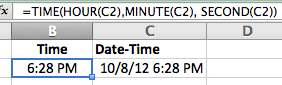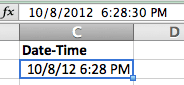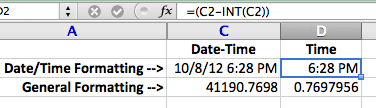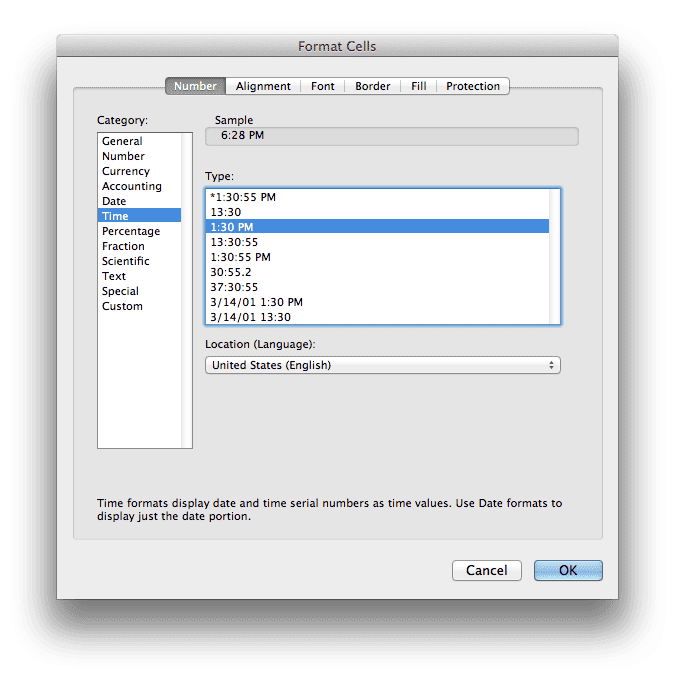# Extract Time from a Date-Time Number in Excel

I have a worksheet that tracks start and stop times for different events throughout the day, all during the week. Sometimes I have to pull out the Time of Day, irrespective of the Date, with the TIME function.

The TIME function has three arguments: Hour, Minute, Second. I could use =TIME(11,30,0) in a cell to get 11:30 AM, but I want to convert a Date-Time number so let’s look at an example.

In cell C2 I have the Date-Time value 10/8/12 6:28:30 PM.In cell B2 I enter the formula =TIME(HOUR(C2),MINUTE(C2), SECOND(C2)) to pull out the time value. Notice that the HOUR, MINUTE, and SECOND functions are used to extract values for the Hour, Minute, and Second arguments. The TIME Function puts these into a nicely formatted time value of 6:28 PM.Now the Time values can be used independently of the Date.

Note: You don’t see the 30 seconds in cell B2 because of the default cell formatting for Time in my spreadsheet.

## A Shortcut Formula [UPDATE]

I had a reader comment about an easier formula so I will include it in this post. Thanks JMarc.

A Date-Time serial number, with General formatting, shows up as an Integer.Fraction. You don’t normally see this on your spreadsheet as Excel will automatically format Date-Time numbers as, well, Date-Time numbers.

The screen shot below shows the Date-Time number and its serial number equivalent with General formatting. Both underlying numbers are identical, the cell formatting is the only difference.The INT function returns the integer portion of a number. If we use the Date-Time number and subtract the integer portion, that leaves us with the fractional portion, which is the Time. The formula =C2-INT(C2) will return the number 0.769795602.

Reformatting the cell to a Time format will allow the fraction to show as a Time value.Changing the cell formatting gives us the time value 6:28 PM.## 12 thoughts on “Extract Time from a Date-Time Number in Excel”

1.JMarc

Hi,
Like in your previous post, you could also use in B2 : = C2 – INT(C2)
and format B2 with Time format.
Shorter formula ! 😉

1.Gregory

Your absolutely right. I was trying to keep my explanation short, hence the omission. Good topic for a follow up post, eh? I’ll even give you the credit and send a link your way.

2.ruve1k

Just use the MOD function to get the fractional portion. =MOD(C2,1)

1.Gregory

THANK YOU for pointing this out!

Honestly, I can’t believe I missed using the MOD Function to pull out the fractional portion of a number.

3.Vanessa

Hi;

Im working on an analysis and need to calculate no. of day and time

like start 1/12/2012 12:57:00 AM and end 4/12/2012 4:32:00 PM. Can u help me with the formula. I want the result as no of day, hh:mm

Regards,
Vanessa

1.Gregory

Assuming Start value in cell A2 = 1/12/2012 12:57:00 AM and End value in cell B2 = 4/12/2012 4:32:00 PM you can use the following formula:

=INT(B2-A2) & ” days, ” & TEXT(MOD(B2-A2,1),”hh:mm”)

that will return 91 days, 15:35 for the answer.

If I switch the times, but leave the same dates we have A2 = 1/12/2012 4:32:00 PM and B2 = 4/12/2012 12:57:00 AM. The formula will return 90 days, 08:25 for the answer. Both answers are correct and the formula is validated.

4.Vanessa

Thanks alot, great help.

5.Anju

Hi,

We work from 9:30 to 6:00.

start Date: 01-dec-2012 4:00 pm
End Date and Time: 15-jan-2013 11:00 am.

help m in getting the formulae

1.Gregory

Put the first number in cell A1 and the second number in cell B1, then in cell C1 use the formula =B1-A1 which will give you the result 34.79 if you format the cell C1 with a General format. That’s 34.79 days.

In cell D1 you can enter the formula =MOD(C1,1)*24 which gives the result 19. That’s 19 hours.

In cell E1 you can enter the formula =INT(B1-A1)&” days, “&TEXT(MOD(B1-A1,1)*24,”#.00″)& ” hours” that will give you the result 34 days, 19.00 hours.

1.Anju

Thanks for the reply…no of hours per day is 8.. how do we calculate it? above we are doing it for 24 hours.. but we need per day 8 hrs.

2.Gregory

Are weekends included, or only weekdays?

1.Anju

Only Week Days…some times we calculate weekends also…

can you please suggest for both..